Understanding Rheology
Oxford University Press, 2001.  Available from Amazon.com

Dr. Faith A. Morrison, Associate Professor of Chemical Engineering
Michigan Technological University
1400 Townsend Drive
Houghton, MI 49931-1295
email fmorriso@mtu.edu

This web page also includes extra problems (see below in red) and extra tables that are not included in the text.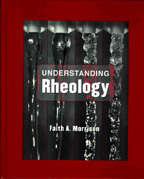Errata for Understanding Rheology

Preface
Chapter 1
Chapter 2
Chapter 3
Chapter 4
Chapter 5
Chapter 6
Chapter 7
Chapter 8
Chapter 9
Chapter 10
Appendix A
Appendix B
Appendix C
Appendix D
Appendix E
Appendix F
References

Preface

p. xi, line 23. Add reference 139 to the list, Ronald G. Larson, "Structure and Properties of Complex Fluids," Oxford, NY, 1999.

Chapter 1

p. 1, line 18; remove reference 61 from the second list of references.

Chapter 2
p. 13, line 8 from bottom. Change “speed of light” to “speed of light in vacuum”.
p. 13, fig. 21. Change “Chapter 4: Standard Fluids” to “Chapter 4: Standard Flows”.
p.16: The vector product is not associative.
p.31 - equation 2.102; there should be two underbars on the tensor A.
p.39, the definition for the tensor magnitude in equation 2.174 is correct, I believe (no transpose); it does not agree with Bird, Armstrong, and Hassager Dynamics of Polymeric Liquids, which says A:AT (page 566, equation A.3-11), but it does agree with the magnitude of the rate-of-deformation tensor in the same book on page 171.  Since the rate-of-deformation tensor is symmetric, the difference of opinion on the definition has no effect in this case.  The definition containing A:A seems preferable to me since it is related to a tensor invariant.  The quantity A:AT is not related to a tensor invariant when the tensor is not symmetric, although it is always real, while the invariant is not guarunteed to be real.  All this not withstanding, the A:AT definition is on the web (see wikipedia); I cannot explain the discrepancy.
p. 52, line 5 from bottom. Change “Table C.7” to “Table C.3”.
p. 53, line 20. Change “Tables C.7 and C.8” to “Tables C.3 and C.4”.
pp. 54-55 Leibnitz should be spelled Leibniz throughout this discussion and in the table of contents for this section.
p.58 - Problem 2.40.  The definition shown is for the second invariant, not the first invariant.  See the definitions on page 40 (equation2.180).

Extra Problems:
2.48  Calculate the invariants of the tensor A given in problem 2.11 using the representation of the tensor A in the 123 coordinate system and the definitions of the invariants given in equations 2.185-2.187 on page 40 of the text.  Also make the same calculation (taking the trace of A, A2 and A3) using using the representation of A in the new coordinate system (results of problem 2.11 caculated above in problem 3).  What do you notice?  Explain. (Ref HW1 2005)
2.49  Do the following vectors form a basis? u=(1, 3, 0)xyz; v=(2, 1, -1)xyz; w=(1, 0, -1)xyz. If yes, write the vector (1, 1, 1)xyz in the basis formed by u, v, and w.   (Ref HW1 2003)
2.50  Write out the product of the two tensors b c . A in Einstein notation, where A is a tensor. What is the "13" component of the transpose of b c . A?  (Ref HW1 2003)

Chapter 3
p. 59, last line. Change “mass in – mass out = 0” to “mass in – mass out = accumulation of mass”.
p.69 - equation 3.58; the tensor Pi should have a double underline.
p. 81, line 4. Remove the space in “d x2”.
p.92 - equation 3.215; the z in the denominator should be L and the term before the plus sign should be muliplied by z.  The equation is essentially the same as equation 7.84 on page 241.
p.99 - Problem 3.7 - Two problems were run together during typesetting.  The first problem ends with the period after viscosity.  The second problem begins "The equivalent pressure . . . " and goes to the end of the problem.  The solution to this second problem is not in the instructor's manual.
p.100 - Problem 3.17 - Calculate the velocity profile and the flow rate per unit width . . .

Extra Problems:
3.26  Beginning with the momentum balance with stress appearing (the Cauchy Momentum equation or Equation of Motion, equation 3.96), derive the Navier-Stokes equation (equation 3.104).  Please use Einstein notation to prove your steps.   (Ref HW3 2003)

Chapter 4
p.109 - Equation 4.8 - the division by 2 should be within the bounds of the square root, i.e. same expression as equation 2.174.
p110 - There is a typesetting problem in the matrix of equation 4.12.
p.116 - Equation 4.28, the left side of the equation should be the scalar gamma dot (no double underlines).
p.118 - Figure 4.12b; on the right side, the lower point should be labeled P2 instead of P1; also in there is a "0" that should be a subscript in the equation for length (left side of figure b).
p.118, last line, partial v with respect to t should be the substantial derivative of vector v, i.e. Dv/Dt.
p.119, Equation 4.42, the first vector quantity should be replaced with the expression for substantial derivative of v, i.e. partial v/partial t + v dot del v.  The second and third vector quantities are correct.
p.119, Equation 4.43, the first vector quantity should be replaced with the expression for substantial derivative of v, i.e. partial v/partial t + v dot del v.  In the second vector quantity, the x1 component of the vector should be v1 partial v1/partial x1.  The final vector quantity is correct (zero vector).
p. 124, last and second-to-last lines in paragraph. Change “coordinates of the stress tensor” to “components of the stress tensor”.
p.128 - Problem 4.6, the word "is" should be "are"; also change “are given.” to “are given below.”

Extra Problems:
4.19  Write out the expression in equation 9.194 (page 336 of the text, the upper-convected derivative) in component form (i.e. using Einstein notation for each vector and tensor in the equation, carry out the dot products.  Note that there is a dot product within the definition of the substantial derivative.  Then collect all the terms together so that they look like a complex scalar coefficient expression multiplied by the basis vectors).  Note that there is a typo in equation 9.194:  the substantial derivative should be DA/Dt (small t not capital T).  (Ref HW3 2005)
4.20  Why do we discuss normal stress differences instead of normal stresses directly?  Why not just do the simpler thing and talk about bare normal stresses?   (Ref HW3 2004)
4.21  Material functions in shear flow are written in terms of t21, t11, t22, and t33. Why are the other five components of the stress tensor not included in definitions of shear material functions?  (Ref HW3 2004)
4.22  Which flow, shear or uniaxial elongation, subjects fluid particles to more severe deformation?  Explain your answer.
4.23  What is the differentce betweeen uniaxial elongation and biaxial elongation?
4.24  Creep is a type of shear flow.  How is creep different from other types of shear flow?

Chapter 5

p. 134. In the sentence after eq. 5.7 change "produced in a rheometer" to "produced near the wall in a rheometer."
p. 135, footnote. Add Husband’s addendum to the Society of Rheology nomenclature. D. M. Husband, Journal of Rheology 36, 409-410 (1992).
p. 157 In equation 5.141 I used a reference time of negative infinity for the strain; that is not correct.  If you use zero for the reference time and then carefully take the real part (see footnote at the bottom of the page), you get the same answer for equation 5.143, however.
p. 167, prob. 5.12. Change “the ratio of the amplitude of the strain 90° out of phase with the amplitude of the stress to the stress” to “the ratio of the amplitude of the strain 90° out of phase with the stress to the amplitude of the stress”.

Extra Problems:

5.19  Why are there so many material functions?  Why not just define steady shear viscosity and be done with it?  Please answer in up to 5 or so sentences.  (Ref HW4 2005)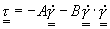where A and B are constant scalar parameters of the model.  Make suggestions on how to improve the model based on what you know of polymer behavior.  (This constitutive equation is a simple version of the Reiner-Rivlin equation; see Dynamics of Polymeric Fluids by Bird, Armstrong, and Hassager, 2nd edition page 504)  (Ref HW4 2003)
5.21  Calculate and sketch (as a function of time) the three material functions of shear stress decay (stress relaxation in Figure 5.2) for the ad-hoc constitutive equation proposed below:where the function M is given by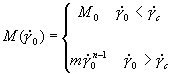and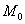, m, n, andare constant scalar parameters of the model.  In your sketch, plot the material functions,  andfor several values of the shear rateas a function of time.  For guidance see the example on page 138 and the sketch in Figure 5.3.   (Ref HW4 2003)

Chapter 6

p.201 - In the last line on this page a hyphen is missing in "Williams-Landel-Ferry".
p.218 - The page number is missing.
p. 222, prob. 6.5 Change "Plot your results." to "Plot your results for the shifted moduli functions and for damping function versus strain amplitude."
p. 222, prob. 6.6 and p. 223, caption to fig. 6.70. Change “two monodisperse polymers” to “two monodisperse polymers of the same type.”

Extra Problems:

6.9  Sketch viscosity versus shear rate and first normal stress coefficient versus shear rate for a typical polymer. Please remember to label your axes (log, or not log, what is being plotted, etc.) . Please indicate any key features on the graph.   (Ref HW5 2004)

Chapter 7
p.227 - Equation 7.1 is for the tensor del v not gamma dot.
p. 232, line 15. Change "the fluid flows with a constant viscosity" to "the viscosity of the fluid approaches a constant."
p. 251, prob. 7.18. Change “complex viscosity” to “dynamic viscosity”.
p. 252, problem 7.24.  A range for plotting is given for omega to vary from 0.001 to 1,000.  It should be a range of gamma_dot, not omega.
p. 230, Figure 7.1, The Newtonian line has gamma-dot-superscript-0; this should not have a superscript-0 but should just be a plain gamma-dot.
p 254.  problem 7.28, part c.  It says to plot the velocity profile as v_x/v_x,av versus h/H.  What is meant is versus y/H, where y is the coordinate direction through the thickness of the fluid.

Extra Problems:
7.36  Will a fluid that follows the power-law generalized Newtonian fluid constitutive equation exhibit rod climbing? Why or why not?  (Exam1 2003)
7.37  For a power-law, generalized Newtonian fluid, solve for the velocity profile for steady flow between wide parallel plates when the upper plate is moving backwards at a velocity V and there is an imposed negative pressure gradient.  The problem is outlined in this pdf file, and the solution is given here.

Chapter 8
p. 273, eq. 8.90. Change “epsilon dot zero > 0) to "epsilon dot (t) > 0".
p. 298, line 3. Change “aide” to “aid”.
p. 300 - Problem 8.4.  In this problem I say "All constitutive equations we have studied so far predict a stress tensor tau proportional to the shear reate tensor gammadot."  This is not strictly correct.  In the power-law model we allow nonlinearites in the form of a viscosity that depends on the magnitude of the gammadot tensor.  What I mean to say is that all of them are something that multiplies the gammadot tensor.
p.303 - Problem 8.28, part b is unclear.  A better wording would be "For a single-relaxation-time Maxwell model and for the actual data discussed in the example in Section 8.3, 1/omega_crossover was approximately equal to the longest relaxation time, lambda_1.  Is this true for the data in this problem?  Is it a good idea to associate 1/omega_crossover with the longest relaxation time in all cases?"
p.303 - Problem 8.31, instead of G' and G' it should read G' and G''.
p.304 - Problem 8.34 Gi should be gi, the parameters of the generalized Maxwell model.
p.304 - Problem 8.34, part a.  You need to choose two parameters.  Two good choices are gi=G=1000Pa and l1=10s.
p.304 - Problem 8.34, part b is unclear.  A better wording would be "At high frequencies (past the terminal regime) what is the slope of logG' vs. log omega and logG'' versus log omega for the full Rouse model?"  For more information on the Rouse model, click here.

Extra Problems:
8.36 For the material that has the linear-viscoelastic spectrum given in problem 8.23, predict the measured shear stress as a function of time for a step strain of 2.  Plot your prediction. (Ref CBE614 HW4)
8.37 Compare and contrast the Generalized Newtonian fluid models of Chapter 7 and the Generalized Linear Viscoelastic models of Chapter 8.  When are each useful?  List three material behaviors (as discussed in Chapter 6) that are correctly captured by these models and list three material behaviors that are not correctly captured by these models. (Ref HW62007)

Chapter 9
p.311 - in equations 9.29, 9.31, and 9.34 the derivatives should be partial derivatives (in 9.31 only the derivative after the dot product is wrong; the dr and dr' are correct).
p.324 - the line after equation 9.120 should be "where gamma = gamma(t,0) = integral from t to 0 gamma^dot_0 dt'' = - gamma^dot_0  t."
p.324 - equation 9.121 after the second equals sign should have a negative sign
p.325 - Figure 9.6 - The line for shear stress should be labeled -tau_21 (the negative sign is missing).
p. 329 - Table 9.3 caption at the bottom.  The angle psi is the angle from r(t')=r' to r(t)=r in counterclockwise rotation around the e_3 axis (not the other way around).
p.333 - equation 9.166; the y(bar) should be y(bar)' (with a prime)
p.336 - equation 9.194; in the substantial derivative the denominator should be "Dt" rather than "DT"
p.351 - equation 9.295; the capital pi should have a wide hat not a small hat and the subscript x should have a small hat not a wide hat.
p.364 - equation 9.329; the stress squared term in the Giesekus equation should be preceded by a negative sign (minus) rather than a plus.
p.367 - The symbol f~ defined in equation 9.333 and used throughout this page is not the same quantity as defined in equation 9.332.  A different symbol should have been used.
p. 368, eq. 9.342. Change “probability a chain of end-to-end vector R crosses surface dA” to “probability the end-to-end vector R crosses surface dA”.
p. 368, line 13. Change “given by the conformation distribution function” to “given by the nonequilibrium configuration distribution function”.
p. 369, eq. 9.344. Change “probability a chain of end-to-end vector R crosses surface dA” to “probability the end-to-end vector R crosses surface dA”.
p.370 - In the first line <RR> should be <R R>.
p. 372, eq. 9.359. Remove the commas.
p. 373, eq. 9.367 and 9.368. Change “probablity” to “probability”.
p. 376, line 9. Change “inverse deformation tensor” to “inverse deformation-gradient tensor”.

Extra Problems:
9.59  The Second-Order Fluid (see page 456) is a non-linear constitutive equation that predicts normal stresses in shear.  The Second-Order Fluid (SOF) is a special case of the Oldroyd 8-constant fluid.  What values of Odroyd's 8 constants will give the version of the SOF shown on page 456? (Ref CBE614 HW5)
9.60  We saw that the elastic dumbbell model predicts the upper-convected Maxwell (UCM) model. We also saw that the Green-Tobolsky temporary network model predicted the UCM model.  How is it possible that two different molecular pictures predict the same constitutive equation? (Ref CBE614 HW5)
9.61  Show that the motion (displacement) of the mechanical system shown in Figure 9.12 is governed by a differential equation that is analogous to the Jeffreys model (equation 9.311).
9.62  What is affine motion? (Ref CBE614 HW5)
9.63  The second-order fluid constitutive equation is given in the Glossary on page 456.  Compare the form of this equation with the other advanced constitutive equations we have discussed in Chapter 9.  What equations is it similar to?  In what way is it different?  (Ref HW7 2005)
9.64  What is the strain tensor associated with the GLVE model?  If we "fix" the GLVE model by replacing the strain tensor, we get a new model, the Lodge Rubberlike Liquid model.  What is "wrong" with the GLVE strain tensor that it needs to be "fixed"?  (Ref HW7 2005)
9.65  What is the generalized linear viscoelastic fluid model (GLVE) useful for?  What are the problems associated with this model?(Ref HW7 2005)
9.66  What molecular-based constitutive equations are the mathematical equivalent of the (fluid-mechanics-based) upper-convected Maxwell model?  Comment on this observation.  (HW7 2004)

Chapter 10
p.391 - Equation 10.44 should be labeled "Wall shear rate in capillary flow of any homogeneous fluid".
p. 393, line 10 from bottom. Change “Q” to “Q/R3”.
p. 435 - The problem should read:  "For the data in Figure 10.8, calculate the true pressure drop as a function of L/R."
p.436 - In problem 10.19 parts b) and c) the shear rate listed should be 10 s-1 (the "s" is missing in both cases)

Extra Problems:
10.37

Appendix A

p. 443. For β, add “9.4.2.1 β2 = 3/(2Na2)”.
p. 445. Add “ψ0(R) 9.4.2.1 equilibrium configuration distribution function” and ψ(R) 9.4.2.1 nonequilibrium configuration distribution function”.

Appendix B

Appendix C
p466 - In equation C.4-8, the componentshould be.
p467 - In Table C6 the equation of motion in cylindrical coordinates is correct for a symmetric stress tensor.  For a stress tensor that is not symmetric, the r- and theta-components must be replaced with the equations below (reference:  Dynamics of Polymeric Fluids by Bird, Armstrong, and Hassager).  The z-component is correct as printed for both case.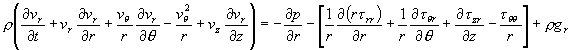(C.6-4)(C.6-5)

p467 - In Table C6 all three components of the equation of motion in spherical coordinates contain errors (reference:  "Dynamics of Polymeric Fluids" by Bird, Armstrong, and Hassager, although as printed it matches Bird Stewart and Lightfoot "Transport Phenomena; I'm still checking).  The correct (according to DPL) components are given below.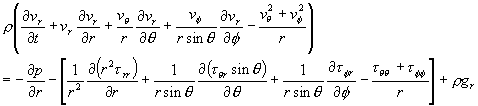(C.6-7)(C.6-8)(C.6-9)

p. 468, eq. C.7-9.  Change "-vf/(r2 sin q)" to "-vf/(r2 sin2q)"
p. 468, last line. Change "nabla" to "nabla squared."

Appendix D

Predictions of GLVE Model, (PDF) posted 24 October 05
Predictions of Corotational Maxwell Model, (PDF) posted 23 November 05
Predictions of Corotational Jeffreys Model, (PDF) posted 23 November 05

Appendix E

Appendix F

References
p. 523, ref. 5a. Change “Matricies” to “Matrices”.
p. 524, ref 21. Change “T. G Fox” to “T. G. Fox”.
p528 - Reference 110, the year should be 1954 not 1964; also Change “Gasses” to “Gases”